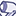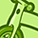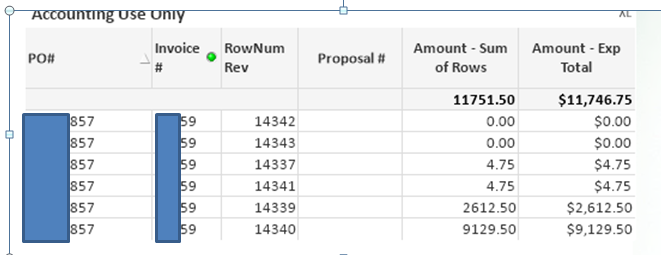# QlikView App Dev

Discussion Board for collaboration related to QlikView App Development.

Announcements
Our next Qlik Insider session will cover new key capabilities. Join us August 11th REGISTER TODAY
cancel
Showing results for
Did you mean:Contributor II

## Total does not match sum of adding individual rows

Has anyone come across an issue in their straight table that the Qlikview calculated total does not match the total if we add the item row by row in Excel?

I'd like to know what could be potential issues and solutions to fix it.

Thanks,

Amit

6 RepliesMVP

That is very strange, would you be able to share a file where you can show the issue?Creator

I'm having an identical issue.

Straight table, dimensions have uniqueness, but the total does not match the sum of rows.

For some reason it is not including some rows in the total when the amounts are identical.

In the below image, the Sum of Rows shows the correct total, but the Expression Total is off by 4.75.

The expression is  sum( DISTINCT NetSalesValue).  The first three columns are the dimensions.edit:  when I remove the Distinct, the total jumps up to 2M, which is also not correct.

Any thoughts?MVP

Is this in a straight table? If it is, then try to set the total mode to Sum of rows on the expression tab. If it is a pivot table, try this

Sum(Aggr(Sum(DISTINCT NetSalesValue), [PO#], [Invoice #], [RowNum Rev]))Specialist

Hi,

Sum of rows actually do sum after each rows.

Expression total do on Total values except dimensions

Regards,

MayankMVP

That "distinct" can change things between rows and totals, if there is  the same NetSalesValue in two or more different rows, it will be counted only once in totals.

Doing as Sunny says it will be counted as it was the sum of different rows (so the same NetSalesValues can be counted more than once)Contributor

I have the same issue.

What is odd is if I add that same column to the straight table as a dimension, it adds the missing lines and then it does add all values correctly.

I am doing a SUM of Rows on my expression.

I will need to leave a duplicate of this column to get the correct total.

This is concerning since I am not sure what other apps already created have this issue!

Any suggestions? Thank you in advance!Community Browser